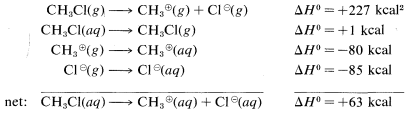# 8.3: Thermochemistry of Substitution Reactions

$$\newcommand{\vecs}{\overset { \rightharpoonup} {\mathbf{#1}} }$$ $$\newcommand{\vecd}{\overset{-\!-\!\rightharpoonup}{\vphantom{a}\smash {#1}}}$$$$\newcommand{\id}{\mathrm{id}}$$ $$\newcommand{\Span}{\mathrm{span}}$$ $$\newcommand{\kernel}{\mathrm{null}\,}$$ $$\newcommand{\range}{\mathrm{range}\,}$$ $$\newcommand{\RealPart}{\mathrm{Re}}$$ $$\newcommand{\ImaginaryPart}{\mathrm{Im}}$$ $$\newcommand{\Argument}{\mathrm{Arg}}$$ $$\newcommand{\norm}{\| #1 \|}$$ $$\newcommand{\inner}{\langle #1, #2 \rangle}$$ $$\newcommand{\Span}{\mathrm{span}}$$ $$\newcommand{\id}{\mathrm{id}}$$ $$\newcommand{\Span}{\mathrm{span}}$$ $$\newcommand{\kernel}{\mathrm{null}\,}$$ $$\newcommand{\range}{\mathrm{range}\,}$$ $$\newcommand{\RealPart}{\mathrm{Re}}$$ $$\newcommand{\ImaginaryPart}{\mathrm{Im}}$$ $$\newcommand{\Argument}{\mathrm{Arg}}$$ $$\newcommand{\norm}{\| #1 \|}$$ $$\newcommand{\inner}{\langle #1, #2 \rangle}$$ $$\newcommand{\Span}{\mathrm{span}}$$$$\newcommand{\AA}{\unicode[.8,0]{x212B}}$$

Ionic or polar reactions of alkyl halides rarely are observed in the vapor phase because the energy required to dissociate a carbon-halogen bond heterolytically is almost prohibitively high. For example, while the heat of dissociation of chloromethane to a methyl radical and a chlorine atom is $$84 \: \text{kcal mol}^{-1}$$ (Table 4-6), dissociation to a methyl cation and a chloride ion requires about $$227 \: \text{kcal mol}^{-1}$$:However, the heat of ionic dissociation of methyl chloride in aqueous solution is estimated to be $$63 \: \text{kcal}$$, and while this reaction is still substantially endothermic, it requires about $$227 - 63 = 164 \: \text{kcal}$$ less energy than in the gas phase:The reason is that ions are much more stable in water than in the gas phase; for example, the transfer of a chloride ion from the gas to water is exothermic by $$-85 \: \text{kcal}$$. The $$\Delta H^\text{0}$$ value for the corresponding transfer of a methyl cation, $$CH_3^\oplus$$, is not known with certainty, but is about $$-80 \: \text{kcal}$$. These ionic solvation energies are clearly large. In contrast, the $$\Delta H^\text{0}$$ for solution of methyl chloride in water is small (about $$1 \: \text{kcal}$$). We can use these data to calculate the heat of ionic dissociation of chloromethane in water:Thermochemical data for the solvation of ions as used in the preceding calculations are difficult to measure and even to estimate. Therefore this kind of calculation of $$\Delta H^\text{0}$$ for ionic reactions involving organic molecules in solution usually cannot be made. As a result, we have considerably fewer possibilities to assess the thermodynamic feasibility of the individual steps of polar reactions in solution than we do of vapor-phase radical processes. Bond energies are not of much use in predicting or explaining reactivity in ionic reactions unless we have some information that can be used to translate gas-phase $$\Delta H^\text{0}$$ values to solution $$\Delta H^\text{0}$$ values.

$$^2$$Calculated from the following data: